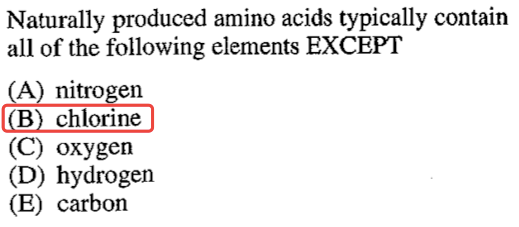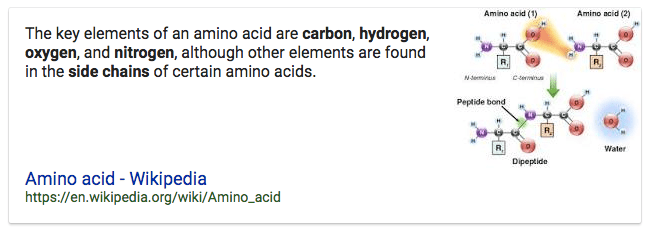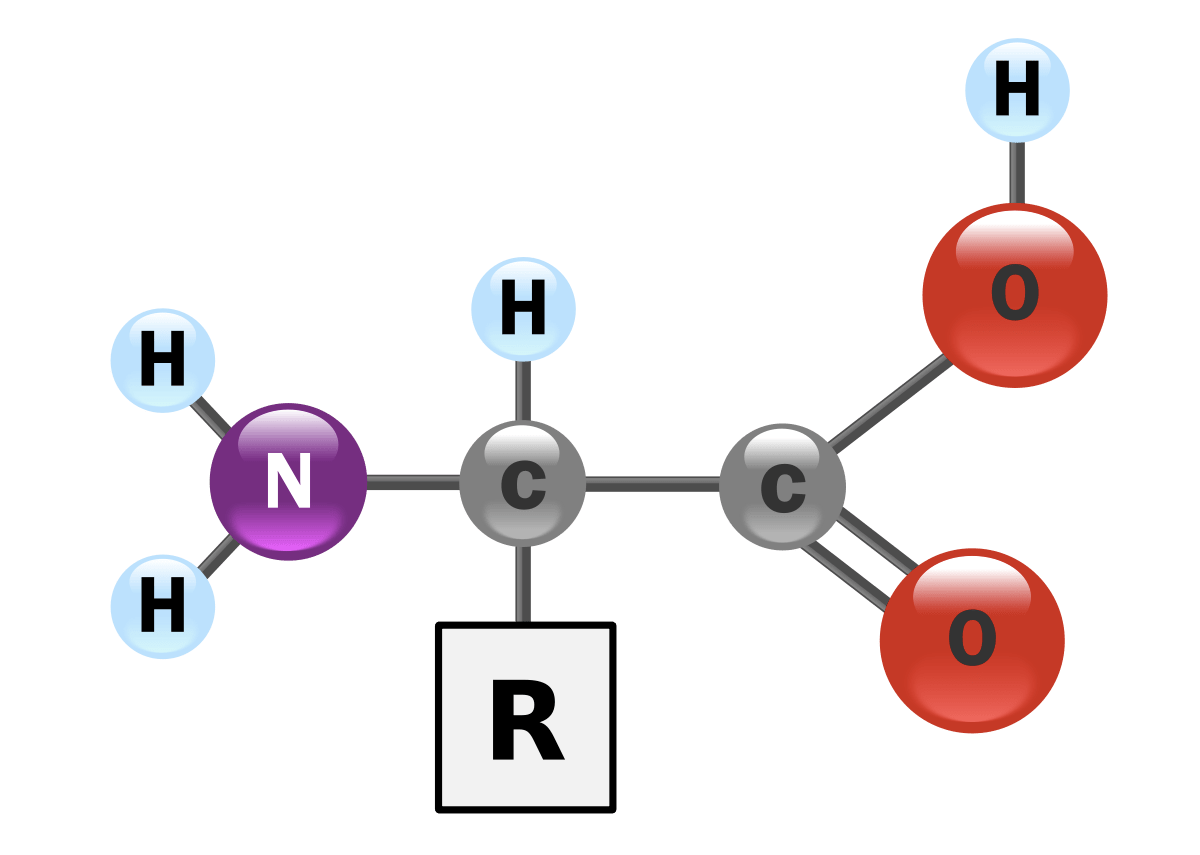# Question 10# Question 11

• Quantity typically determined by measuring the rate of a reaction at two or more different temperatures: Activation Energy

• The activation energy can also be found algebraically by substituting two rate constants (k1, k2) and the two corresponding reaction temperatures (T1, T2) into the Arrhenius Equation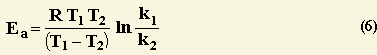• Arrhenius Equation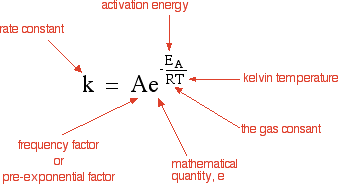# Question 49

• Most Phosphates are insoluble# Question 54• Raoult's law# Question 66• Collision Theory

![Frequency Factor: Number of Collisions per Second Determined by the concentration of the reactants. [Temperature has a smaller influence.) ](./media/image60.png)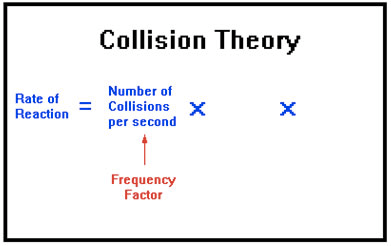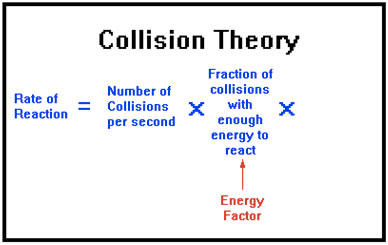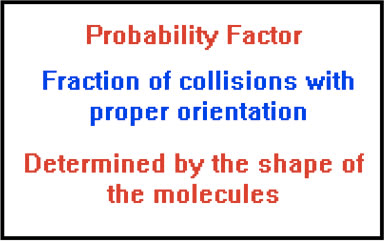# Question 70